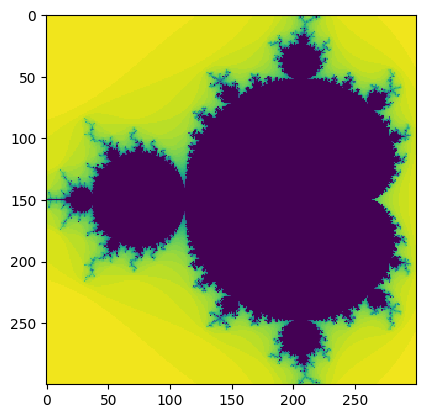Close

COMP0023: Research Software Engineering With Python

HomeIn :
xmin = -1.5
ymin = -1.0
xmax = 0.5
ymax = 1.0
resolution = 300
xstep = (xmax - xmin) / resolution
ystep = (ymax - ymin) / resolution
xs = [(xmin + (xmax - xmin) * i / resolution) for i in range(resolution)]
ys = [(ymin + (ymax - ymin) * i / resolution) for i in range(resolution)]

In :
def mandel1(position, limit=50):
value = position
while abs(value) < 2:
limit -= 1
value = value**2 + position
if limit < 0:
return 0
return limit

In :
data1 = [[mandel1(complex(x, y)) for x in xs] for y in ys]


## Many Mandelbrots¶

Let's compare our naive python implementation which used a list comprehension, taking 662ms, with the following:

In :
%%timeit
data2 = []
for y in ys:
row = []
for x in xs:
row.append(mandel1(complex(x, y)))
data2.append(row)

618 ms ± 1.89 ms per loop (mean ± std. dev. of 7 runs, 1 loop each)

In :
data2 = []
for y in ys:
row = []
for x in xs:
row.append(mandel1(complex(x, y)))
data2.append(row)


Interestingly, not much difference. I would have expected this to be slower, due to the normally high cost of appending to data.

In :
from matplotlib import pyplot as plt
%matplotlib inline
plt.imshow(data2, interpolation='none')

Out:
<matplotlib.image.AxesImage at 0x7f9c03f8aaf0>We ought to be checking if these results are the same by comparing the values in a test, rather than re-plotting. This is cumbersome in pure Python, but easy with NumPy, so we'll do this later.

Let's try a pre-allocated data structure:

In :
data3 = [[0 for i in range(resolution)] for j in range(resolution)]

In :
%%timeit
for j, y in enumerate(ys):
for i, x in enumerate(xs):
data3[j][i] = mandel1(complex(x, y))

612 ms ± 247 µs per loop (mean ± std. dev. of 7 runs, 1 loop each)

In :
for j, y in enumerate(ys):
for i, x in enumerate(xs):
data3[j][i] = mandel1(complex(x, y))

In :
plt.imshow(data3, interpolation='none')

Out:
<matplotlib.image.AxesImage at 0x7f9c03ea0a00>Nope, no gain there.

Let's try using functional programming approaches:

In :
%%timeit
data4 = []
for y in ys:
bind_mandel = lambda x: mandel1(complex(x, y))
data4.append(list(map(bind_mandel, xs)))

612 ms ± 1.31 ms per loop (mean ± std. dev. of 7 runs, 1 loop each)

In :
data4 = []
for y in ys:
bind_mandel = lambda x: mandel1(complex(x, y))
data4.append(list(map(bind_mandel, xs)))

In :
plt.imshow(data4, interpolation='none')

Out:
<matplotlib.image.AxesImage at 0x7f9c01d9bf10>That was a tiny bit slower.

So, what do we learn from this? Our mental image of what code should be faster or slower is often wrong, or doesn't make much difference. The only way to really improve code performance is empirically, through measurements.